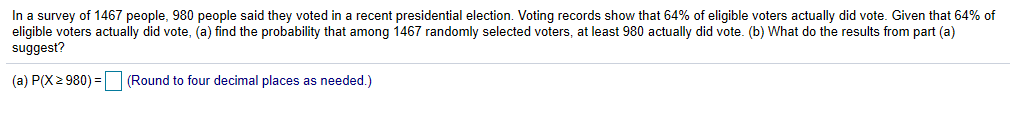# In a survey of 1467 people, 980 people said they voted in a recent presidential election. Voting records show that 64% of eligible voters actually did vote. Given that 64% ofeligible voters actually did vote, (a) find the probability that among 1467 randomly selected voters, at least 980 actually did vote. (b) What do the results from part (a)suggest?(a) P(X 2 980)-(Round to four decimal places as needed.)

Questionhelp_outlineImage TranscriptioncloseIn a survey of 1467 people, 980 people said they voted in a recent presidential election. Voting records show that 64% of eligible voters actually did vote. Given that 64% of eligible voters actually did vote, (a) find the probability that among 1467 randomly selected voters, at least 980 actually did vote. (b) What do the results from part (a) suggest? (a) P(X 2 980)-(Round to four decimal places as needed.) fullscreen
check_circleExpert Solution
Step 1

Let X be the number of person who actually did vote.

The total number of people is 1467.

The voting record shows that 64% di vote.

That is the success event is voted and failure event is not voted.

The assumptions for binomial distribution:

• The number of trials is fixed.
• There are two outcomes success and failure with success probability p.
• Each of the trials will be independent.

Here, all the three assumptions are satisfied, therefore it can be said that X follows binomial distribution

with n = 1,467, p = 0.64.

Step 2

The pmf of binomial distribution is,

Step 3

a.

The probability that at least 980 actually di...

### Want to see the full answer?

See Solution

#### Want to see this answer and more?

Solutions are written by subject experts who are available 24/7. Questions are typically answered within 1 hour*

See Solution
*Response times may vary by subject and question
Tagged in

### Statistics